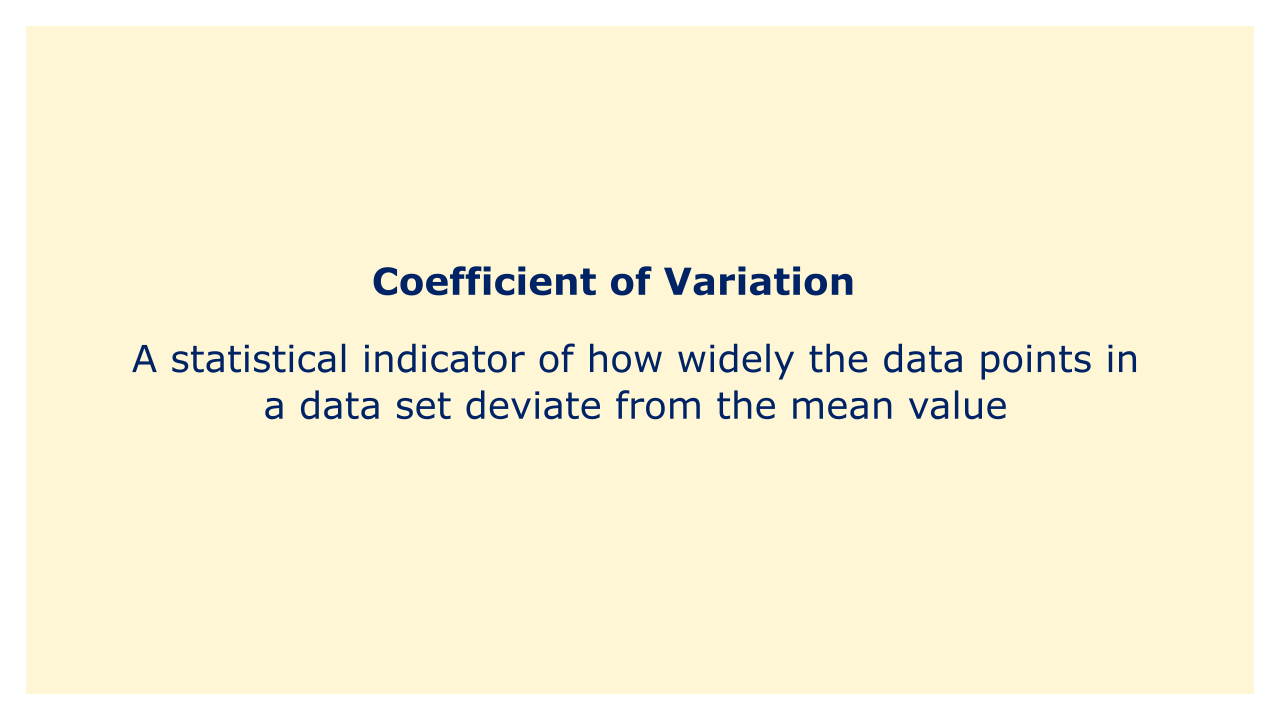# Coefficient of VariationImage: Moneybestpal.com

### The coefficient of variation (CV) is a statistical indicator of how widely the data points in a data set deviate from the mean value. To acquire a percentage, divide the data's standard deviation by its mean value and multiply the result by 100.

The standard deviation is a measurement of how far the data points vary from the mean, while the mean is the average value of the data. Since the CV is a dimensionless quantity, it is independent of the units used to quantify the data. This makes it useful for comparing the variability of data sets with various units or scales.

When comparing an investment's risk and volatility to its anticipated return, the CV is utilized in finance. Investment risk is the degree to which future returns are unclear or variable, whereas investment volatility is the degree to which those returns fluctuate over time. The expected return is the mean or average of the returns that the investor expects to receive from the investment. The CV details the amount of risk the investor is assuming in relation to each unit of predicted return. A lower CV indicates that the investment will have less volatility and more consistency for the same amount of return, which improves the risk-return trade-off.

An investor would choose the investment with a lower CV, for instance, if two investments had the same expected return of 10% but one had a CV of 20% and the other had a CV of 40%. This is because the investment with the lower CV had less risk and more consistency for the same return. By standardizing their risk levels, the CV can also be used to compare other investment kinds, such as equities, bonds, or mutual funds.

The investor can compare the CVs to determine whether an investment has more relative variability for its return, for example, if a stock has an expected return of 15% and a CV of 30% and a bond has an expected return of 5% and a CV of 10%. In this situation, the stock has a higher CV than the bond, indicating greater risk and volatility.
Tags